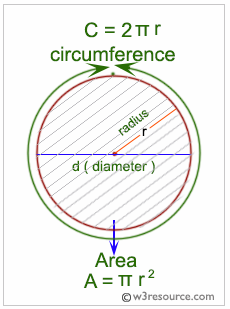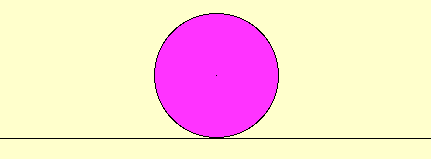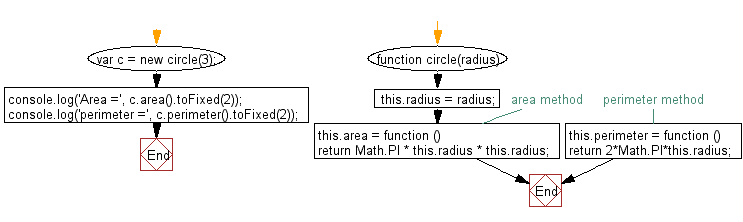# JavaScript: Calculate the area and perimeter of a circle

## JavaScript Object: Exercise-9 with Solution

Write a JavaScript program to calculate the area and perimeter of a circle. Create two methods to calculate the area and perimeter. The radius of the circle will be supplied by the user.

JavaScript: Area and circumference of a circle

In geometry, the area enclosed by a circle of radius r is πr2. Here the Greek letter π represents a constant, approximately equal to 3.14159, which is equal to the ratio of the circumference of any circle to its diameter.

The circumference of a circle is the linear distance around its edge.Why is the area of a circle of a circle pi times the square of the radius?Sample Solution: -

HTML Code:

``````<!DOCTYPE html>
<html>
<meta charset="utf-8">
<title>Area and Perimeter of a circle</title>
<body>
</body>
</html>
```
```

JavaScript Code:

``````function circle(radius)
{
// area method
this.area = function ()
{
};
// perimeter method
this.perimeter = function ()
{
};
}
var c = new circle(3);
console.log('Area =', c.area().toFixed(2));
console.log('perimeter =', c.perimeter().toFixed(2));
```
```

Sample Output:

```Area = 28.27
VM1407:17 perimeter = 18.85
```

Flowchart:Live Demo:

See the Pen javascript-object-exercise-9 by w3resource (@w3resource) on CodePen.

Improve this sample solution and post your code through Disqus

What is the difficulty level of this exercise?

Test your Programming skills with w3resource's quiz.

﻿

## JavaScript: Tips of the Day

How to remove a specific item from an array?

Find the index of the array element you want to remove using indexOf, and then remove that index with splice.

The splice() method changes the contents of an array by removing existing elements and/or adding new elements.

```const array = [2, 5, 9];

console.log(array);

const index = array.indexOf(5);
if (index > -1) {
array.splice(index, 1);
}

// array = [2, 9]
console.log(array);```

The second parameter of splice is the number of elements to remove. Note that splice modifies the array in place and returns a new array containing the elements that have been removed.

For the reason of completeness, here are functions. The first function removes only a single occurrence (i.e. removing the first match of 5 from [2,5,9,1,5,8,5]), while the second function removes all occurrences:

```function removeItemOnce(arr, value) {
var index = arr.indexOf(value);
if (index > -1) {
arr.splice(index, 1);
}
return arr;
}

function removeItemAll(arr, value) {
var i = 0;
while (i < arr.length) {
if (arr[i] === value) {
arr.splice(i, 1);
} else {
++i;
}
}
return arr;
}
//Usage
console.log(removeItemOnce([2,5,9,1,5,8,5], 5))
console.log(removeItemAll([2,5,9,1,5,8,5], 5))
```

Ref: https://bit.ly/2N9nKRp